Hi, I’m Bea Stollnitz, and I’m a principal developer advocate at Microsoft, focusing on Azure ML.

All code in this blog is made available under the MIT license.

• Azure ML

• Creating managed online endpoints in Azure ML

September 10, 2021
In this post, you’ll learn how to create endpoints on Azure that enable real-time predictions using your custom model. I assume familiarity with machine learning concepts, such as training and prediction, but no knowledge of Azure.• Creating batch endpoints in Azure ML

September 13, 2021
In this post, you’ll learn how to create endpoints on Azure that enable asynchronous batch predictions using your custom model. I assume familiarity with machine learning concepts, such as training and prediction, but no knowledge of Azure.• Machine learning frameworks

• Introduction to PyTorch

June 21, 2021
This post introduces PyTorch concepts through the creation of a basic neural network using the Fashion MNIST dataset as a data source. I assume that you have a basic conceptual understanding of neural networks, and that you’re comfortable with Python, but I assume no knowledge of PyTorch.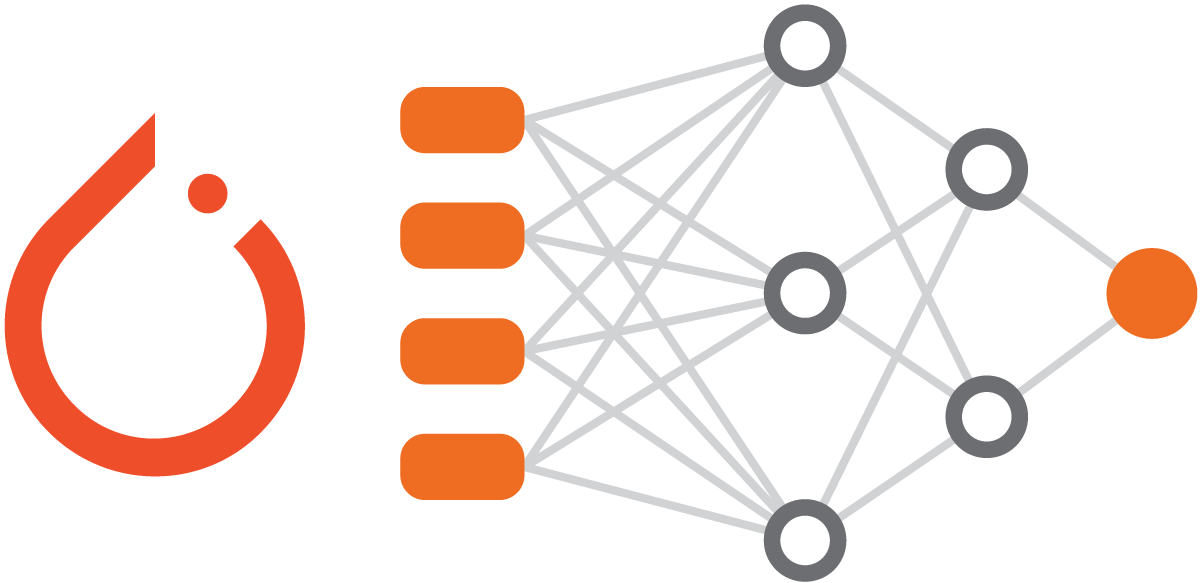• Introduction to TensorFlow using Keras

August 27, 2021
This post provides all the concepts and practical knowledge you need to get started with TensorFlow. We’ll explore Keras, a high-level API released as part of TensorFlow, and we’ll use it to build a basic neural network using the Fashion MNIST dataset as a data source. I assume that you have a basic conceptual understanding of neural networks, and that you’re comfortable with Python, but I assume no knowledge of TensorFlow or Keras.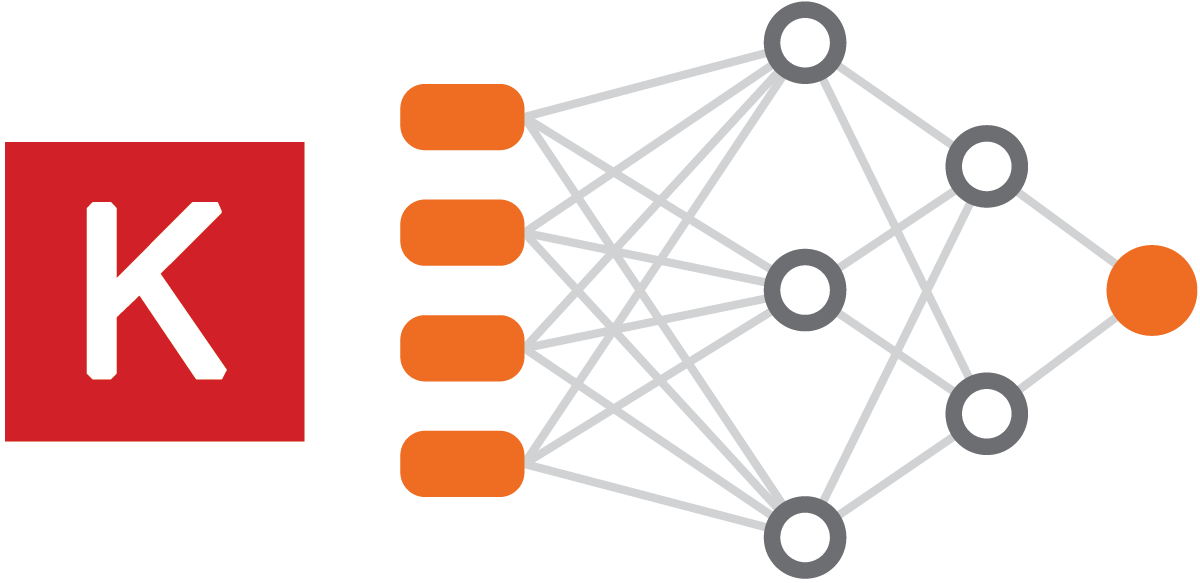• Going beyond Keras - customizing with TensorFlow

September 3, 2021
In this blog post, we’ll re-implement parts of the code from my earlier Keras post, but this time we’ll use lower-level TensorFlow concepts. I assume that you completed my tutorial on Keras or that you have a solid knowledge of Keras, but I assume no knowledge of TensorFlow.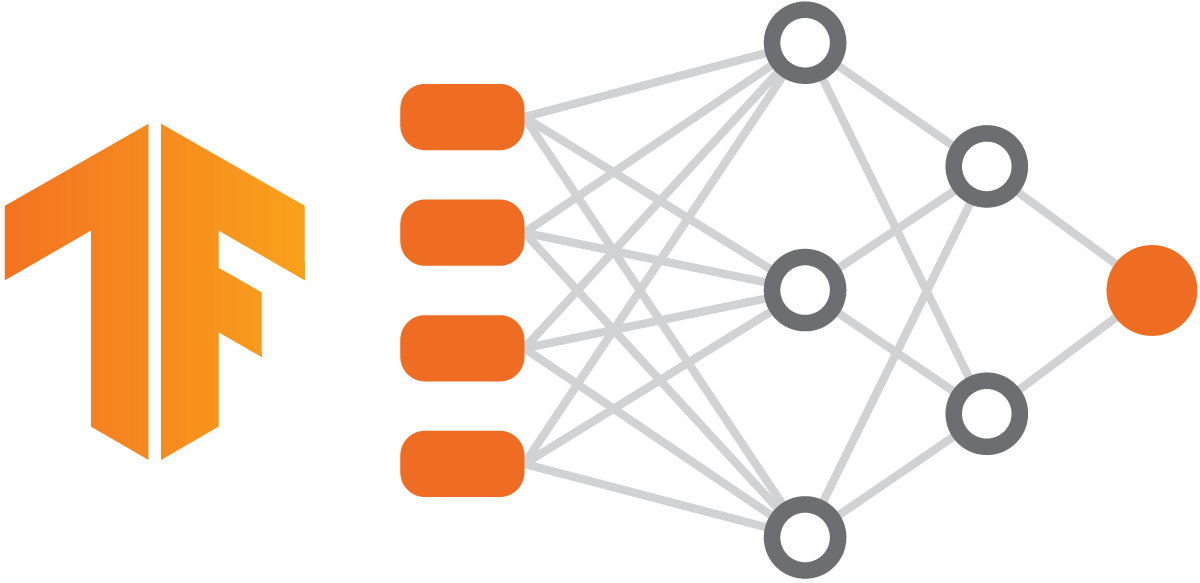• Comparing PyTorch and TensorFlow implementations

September 8, 2021
How do PyTorch code and TensorFlow code compare? Maybe you’re in the beginning phases of your machine learning journey and deciding which framework to embrace, or maybe you’re an experienced ML practicioner considering a change of framework. Either way, you’re in the right place. Drawing from my previous posts, I’ll compare the PyTorch and TensorFlow versions of the code used to classify images in the Fashion MNIST dataset.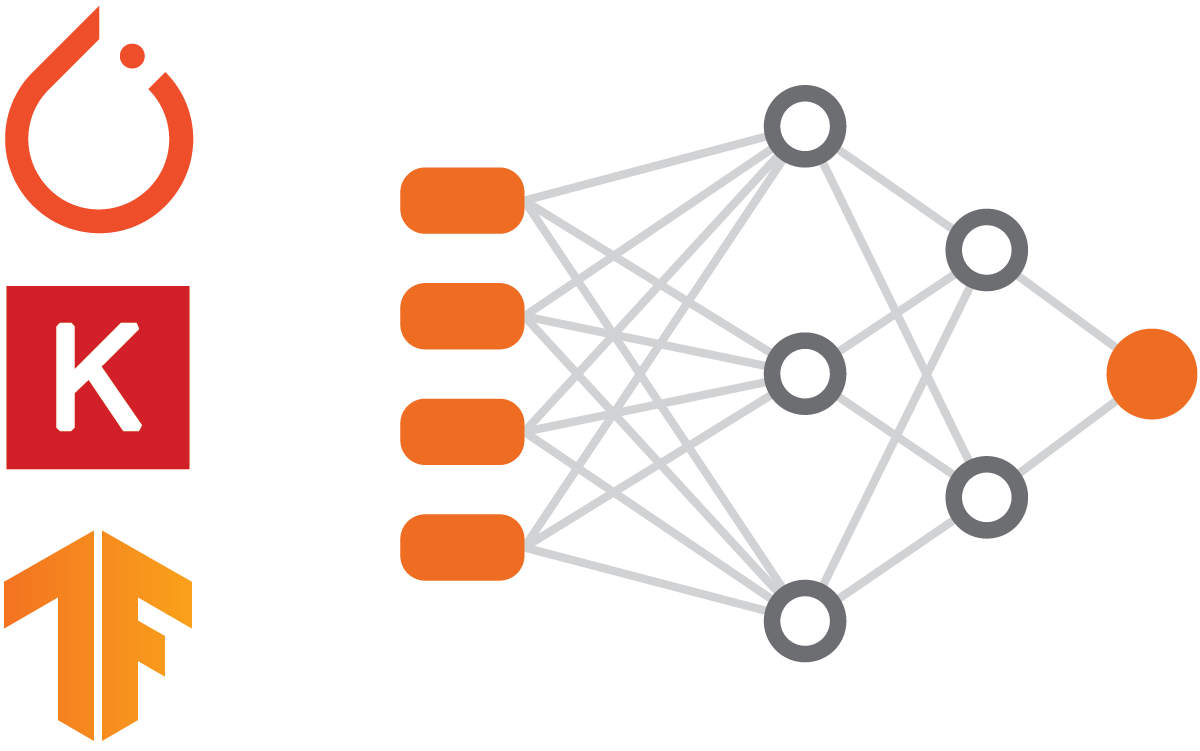• Using PySINDy to discover equations from experimental data

December 30, 2021
In this post, I will use the PySINDy Python package to discover a system of ordinary differential equations that best represents my experimental data. I assume that you read my post “Discovering equations from data using SINDy,” and that you have basic familiarity with ordinary differential equations and dynamical systems.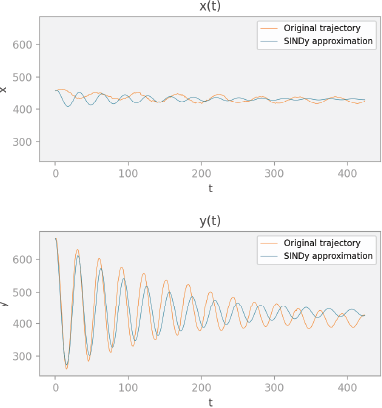• Machine learning techniques

• Discovering equations from data using SINDy

December 29, 2021
In this post, I’ll discuss the 2016 paper “Discovering Governing Equations from Data by Sparse Identification of Nonlinear Dynamical Systems” by Brunton et al. I’ll explain the main concepts of the paper in an accessible way, and I’ll show how we can use its novel approach to discover the Lorenz system of equations from data. I assume basic familiarity with ordinary differential equations and dynamical systems.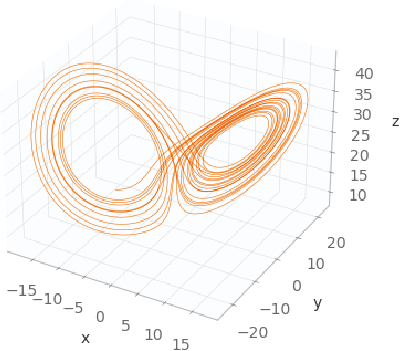• Creating spectrograms and scaleograms for signal classification

January 21, 2022
In this post, I’ll explain how to convert time-series signals into spectrograms and scaleograms. In a future post, we’ll use the images created here to classify the signals. I assume that you have basic math skills and are familiar with basic machine learning concepts.